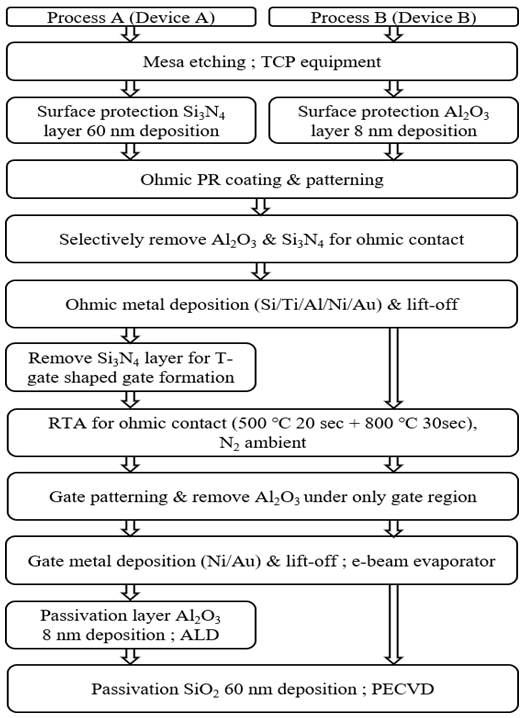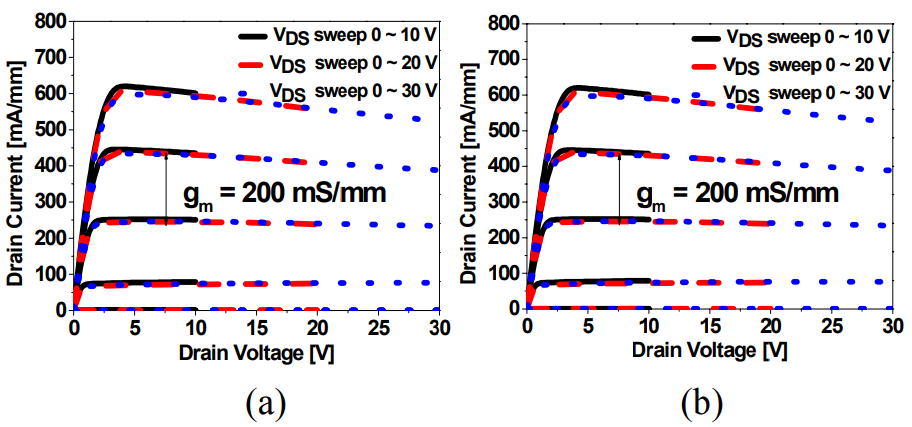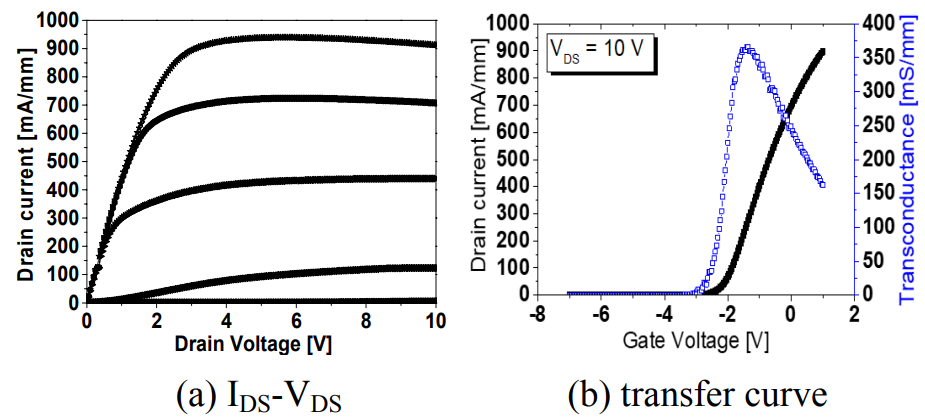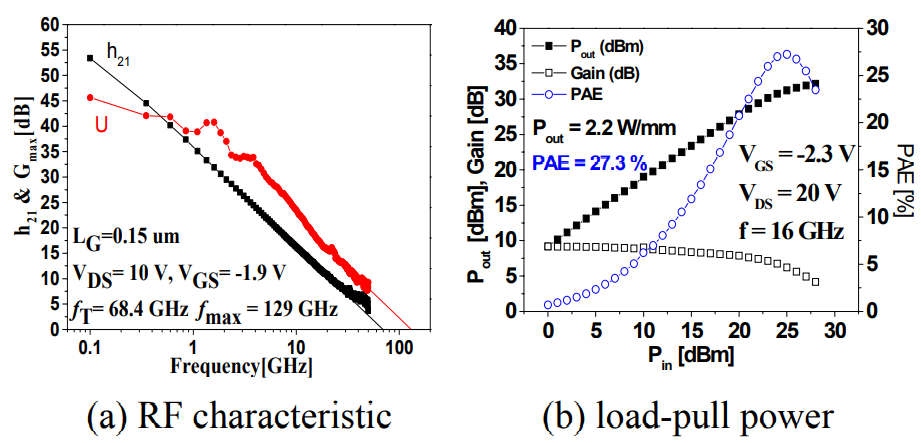Mobile QR Code1. (School of Electronic and Electrical Engineering, Kyungpook National University, Daegu, Korea)

Thin AlGaN layer, Al2O3 surface protection layer, current collapse, RF performance

## I. INTRODUCTION

GaN-based high-electron-mobility transistors (HEMTs) have shown great potential for high-frequency and high-power, and high temperature applications, because they have superior material properties such as high electron mobility, high 2DEG density, high saturation velocity, large band gap energy, and high breakdown field (1-3). However, they still suffer from surface-related problem such as current collapse phenomena, which not only impede device reliability but also degrade power efficiency in GaN-based devices (4-6). To improve the RF performance for millimeter-wave application, scaling down device dimensions, i.e., reducing both the gate length (L$_{\mathrm{G}}$) and the AlGaN barrier thickness is required along with high 2DEG channel mobility and sheet carrier density. As the thickness of the AlGaN barrier decreases, however, the 2DEG properties becomes degraded after high temperature annealing for ohmic contact (7). Therefore, a surface protection layer is usually required to protect the AlGaN surface from the thermal damage during rapid thermal process (RTP). And although several plasma treatments have been recently reported showing that the use of NH$_{3}$ (8), O$_{2}$ + CF$_{4}$ (9), and O$_{2}$/SF$_{6}$ (10) plasma pretreatments prior to passivation could improve device performance. However, in the treatment process, there is a difficulty to take a step to be added, a plurality of optimized conditions. In this work, we present a new structure of protecting the surface of AlGaN/GaN HEMT by using fin thin Al$_{2}$O$_{3}$ layer instead of relatively thick conventional Si$_{3}$N$_{4}$ or SiO$_{2}$ layer, which not only improves the device performance, but also simplifies the fabrication process step.

## II. EXPERIMENT

The AlGaN/GaN heterostructure samples were grown using metal organic chemical vapor deposition (MOCVD) on semi insulating SiC substrate. The epitaxial structures consisted of 60 nm-thick AlN buffer, 2 ${\mathrm{\mu}}$m-thick semi-insulating GaN buffer/channel layer, Si modulation-doped 13 nm-thick AlGaN barrier layer, and 3 nm-thick GaN capping layer was sequentially. The hall mobility and sheet carrier density of the samples were approximately 2100 cm$^{2}$/Vs and 0.9 ${\times}$ 10$^{13}$ /cm$^{2}$, respectively.

Fig. 1. The fabrication of HEMT device structure using SiN protection layer with Al2O3 +SiO2 passivation (process A) and Al2O3 protection layer with SiO2 passivation (process B) interconnection process flow.For the device fabrication, as shown in Fig. 1, two different surface protection techniques were applied to prevent the AlGaN surface from being damaged during high temperature RTP for ohmic contact; surface protection with 60 nm-thick Si$_{3}$N$_{4}$ layer (process A) and 8-nm thick Al$_{2}$O$_{3}$ layer proposed in this work (process B). In this work, double passivation layers, which consists of an 8 nm-thick Al$_{2}$O$_{3}$ layer deposited at 450 ℃ using atomic layer deposition system and a 60 nm-thick SiO$_{2}$ layer deposited by plasma-enhanced chemical vapor deposition, were used to passivate the device surface. The dielectric constant of Al$_{2}$O$_{3}$, Ɛ = 9.12, is larger than that of Si$_{3}$N$_{4}$ and SiO$_{2}$ and comparable with other ‘high-k’ materials. On the other hand, there is no need to remove the thin Al$_{2}$O$_{3}$ layer in process B for the gate formation.

Fig. 2. Typical IDS-VDS curves for the device with 60 nm thick SiO2 passivation on device A (a) and B (b) are Drain characteristics for a HEMT. The drain characteristics indicated by the solid lines are for VDS restricted to < 10 V, the dashed line are for VDS restricted to 20 V and the dot lines are for VDS up to 30 V.The Al$_{2}$O$_{3}$ layer under the gate can be easily removed just by simply increasing the etch time for the gate recess, which not only simplifies the process step, but also effectively protects the device surface during the device fabrication. All other fabrication processes are same for the process A and B. For the ohmic contact, two-step RTP for both processes was carried out initially at low temperature of 500 $^{\circ}$C (20 sec) and then at higher temperature of 800 $^{\circ}$C (30 sec) in nitrogen ambient. The resistance parameters extracted from transmission-line measurement exhibited a low contact resistivity of 2.8 ${\times}$ 10$^{-6}$ Ω-cm$^{2}$ (contact resistance of 0.3 Ω-mm) and the sheet resistance of 318 Ω/${\square}$, almost same results for both process A and B. It is noticed that the sheet resistance for process A was not increased even after high temperature RTP, which indicates that the 8 nm thick-Al$_{2}$O$_{3}$ layer is also very effective for protecting the AlGaN surface as the thick Si$_{3}$N$_{4}$ layer. Ni/Au gate metal with gate length of 2 μm was deposited on the gate region by E-beam evaporator. It was confirmed in Fig. 2 that the current collapse has been improved by using process B. In order to further explore the RF performance, by using process B, we fabricated AlGaN/GaN HEMT with T-gate which has a gate length of 0.15 ${\mathrm{\mu}}$m.

## III. RESULTS AND DISCUSSIONS

Fig. 2 shows comparison of I$_{\mathrm{DS}}$-V$_{\mathrm{DS}}$ characteristics for both devices A and B under various drain-stress voltages after SiO$_{2}$ deposition on the thin Al$_{2}$O$_{3}$ layer. The solid, dashed, and dotted lines correspond to I -V sweeps with the initial drain stress V$_{\mathrm{DS0}}$ of 10, 20, and 30 V, respectively. As shown in Fig. 2(a), device A exhibits reduced drain-stress-dependent current collapse after 60 nm-thick SiO$_{2}$ deposition, but the collapse still exists with apparently larger knee voltage compared to that of the device B. A transconductance (gm) was about 190 and 200 mS/mm for device A and B, respectively. Threshold voltages of both devices are almost same, but device B exhibits much better DC characteristics such as higher transconductance (g$_{\mathrm{m}}$), larger maximum drain current, and better pinch-off with lower knee voltage. This clearly indicates that the Al$_{2}$O$_{3}$ layer in device B becomes dense during high temperature ohmic RTP and well combined with the surface of the GaN capping layer to effectively reduces the trap density at the surface of the device. On the other hand, the Al$_{2}$O$_{3}$ layer deposited after gate metallization in device A does not experience high temperature anneal and is loosely combined with the GaN surface which is not effective in passivating the surface. The reduced current collapse after deposition of relatively thick SiO$_{2}$ is believed to be due to the decreased electron injection into the surface between the gate and the drain electrode. The gradual reduction in current for V$_{\mathrm{DS}}$ > 7 V is attributed to self-heating effect. For device B, the current collapse is not significant as shown in Fig. 2(b), and also showing the self-heating effect. The I-V characteristic and transfer curve of this device are shown in Fig. 3, respectively. The device exhibited a maximum drain current of 943 mA/mm and maximum transconductance (g$_{\mathrm{m}}$) of 370 mS/mm at a gate bias of -2 V and a drain bias of 10 V. The device showed excellent pinch-off characteristics at V$_{\mathrm{GS}}$ = -3 V. As shown in Fig. 3(a), there is no decrease of drain current at high gate and drain voltage induced by self-heating effect because SiC substrate has high thermal conductivity. Fig. 4(a) shows the short circuit current gain (h$_{21}$) versus frequency for this fabrication process device after final SiO$_{2}$ passivation. An extrinsic current gain cut-off frequency (f$_{\mathrm{T}}$) was about 68.4 GHz, the maximum frequency of oscillation (f$_{\mathrm{max}}$) was about 129~GHz. As shown in Fig. 4(b), the maximum output power and the power added efficiency (P.A.E) of this device was 2.2 W/mm and 27.3 % at frequency of 16~GHz, respectively, at a gate bias of -2.3 V and a drain bias of 20 V. As shown in the figure, device apparently demonstrates good RF and load-pull performance because the thin Al$_{2}$O$_{3}$ layer deposited before ohmic RTP is very effective in reducing the surface trap density in the device as discussed above.

Fig. 3. (a) IDS-VDS Characteristics, (b) the transfer curve of the fabricated HEMT.Fig. 4. (a) RF result, (b) load-pull data of the fabricated AlGaN/GaN HEMT.## IV. CONCLUSIONS

We demonstrated the effect of thin Al$_{2}$O$_{3}$ surface protection layer on improving the device performance of AlGaN/GaN HEMTs. The Al$_{2}$O$_{3}$ layer, which was deposited before ohmic RTP, greatly reduces surface trap density which results in the improved current collapse. The use of the Al$_{2}$O$_{3}$ surface protection layer also simplifies device process steps in comparison with conventional SiO$_{2}$ or Si$_{3}$N$_{4}$ protection layer. The device with gate length of 0.15 ${\mathrm{\mu}}$m exhibited high transconductance of 365 mS/mm and drain current of ~943 mA/mm at V$_{\mathrm{GS}}$ = 1 V. The device also exhibited very high RF performances such as f$_{\mathrm{T}}$ and f$_{\mathrm{max}}$ of 67.8 and 125 GHz with P$_{\mathrm{out}}$ of 2.2 W/mm and P.A.E. of 27.3%, respectively.

### ACKNOWLEDGMENTS

This study was supported by the BK21 Plus project funded by the Ministry of Education, Korea (21A20131600011) and the Civil-Military Technology Cooperation Program under Grant (17-CM-MA-03).

### REFERENCES

1
Kahn M. A., Q.Chen , Shur M. S., Mcdermott B. T., July 1996, Microwave operation of GaN/AlGaN-doped channel hetro-structure field effect transistors, IEEE Electron. Device Lett., Vol. 17, pp. 325-3272
Wu Y. F., Kapolnek D., Ibbetson J. P., Parikh P., Keller B. P., Mishra U. K., 2001, Very-high power density AlGaN/GaN HEMTs, IEEE Trans. Electron. Device, pp. 586-5903
Wu Y. F., Keller B. P., Keller S., Kapolnek D., Kozodoy P., DenBaars S. P., Mishra U. K., 1996, Very high breakdown voltage and large transcon-ductance realized on GaN heterojunction field effect transistors, Appl. Phys. Lett., Vol. 69, pp. 14384
Binari S., Ikossi K., Roussos J. A., Kruppa W., Park D., Dietrich H. B., Koleske D. D., Wickenden A. E., Henry R. L., 2001, Trapping effects and microwave power performance in AlGaN/GaN HEMTs, IEEE Trans. Electron. Device, Vol. 48, pp. 465-4715
Vetury R., Zhang N. Q., Keller S., Mishra U. K., 2001, The impact of surface states on the DC and RF characteristics of AlGaN/GaN HFETs, IEEE Trans. Electron. Device, Vol. 48, pp. 560-5666
Tarakji A., Simin G., Ilinskaya N., Hu X., A , Kumar , Koudymov A., Yang J., Khan M. A., 2001, Mechanism of radio-frequency current collapse in GaN–AlGaN field-effect transistors, Appl. Phys. Lett., Vol. 78, pp. 21697
Shijima K., Shigekawa N., 2004, Thermal Stability of Electrical Properties in AlGaN/GaN Hetero-structures., Jpn. J. Appl. Phys., Vol. 43, No. 1, pp. 100-1058
Edwards A.P., Mittereder J. A., Binari S. C., Katzer D. S., Storm D. F., Roussos J. A., Apr 2005, Improved reliability of AlGaN/GaN HEMTs using and NH3 plasma treatment prior to SiN passivation, IEEE Electron Device Lett., Vol. 26, No. 4, pp. 225-2279
Guhel Y., Boudart B., Vellas N., Gaquiere C., Delos E., Ducatteau D., Bougrioua Z., Germain M., Oct 2005, Impact of plasma pre-treatment before SiNx passivation on AlGaN/GaN HFETs electrical traps., Solid State Electron., Vol. 49, No. 10, pp. 1589-159410
Meyer D. J., Flemish J. R., Redwing J. M., may 14/15, 2007, Plasma surface pretreatment effects on Silicon nitride passivation of AlGaN/GaN HEMTs, in Proc. CS MANTECH Conf., pp. 305-307## Author

Ryun-Hwi Kim received the B.S. degree in the Department of Semiconductor Engineering from Ui-duk University, Korea, in 2010 and M.S. degree in School of Electronics Engineering from Kyungpook Na-tional University, Daegu, in 2013 respectively.

He is currently pursuing the Ph.D. degree in the School of electronic and electrical engineering from Kyungpook National University, Daegu.

His interests include GaN-based RF and power device.

Seung-Hyeon Kang received the B.S., M.S., degree in School of Electronics Engineering from Kyungpook National University, Daegu, in 2016 and 2018, respec-tively.

He is currently pursuing the Ph.D. degree in the School of electronic and electrical engineering from Kyungpook National University, Daegu, Korea.

His interests include Growth of nitride-based materials

Bong-Yeol Choi received the B.S. degree in electronic engineering from Kyungpook National University, Daegu, in 1983 and he received the M.S., and Ph.D. degree in electrical and computer engineering from Korea Advanced Institute of Science and Technology(KAIST) in 1985 and 1993.

His research interests include inverse problem, signal processing, image restoration.

Jung-Hee Lee received the B.S. and M.S. degree in electronic engineering from Kyungpook National University, Daegu, in 1979 and 1983, and he received the M.S. degree in electrical and computer engineering from Florida Instituted of Technology in 1986.

He received the Ph.D. degree in electrical and computer engineering form North Carolina State University in 1990.

His current work is focused on growth of nitride-based materials, and Gallium Nitride-based electronic devices.